Name:    Chapter 1 Pre Test

Multiple Choice
Identify the choice that best completes the statement or answers the question.

1.

Determine which of the numbers in the set below are rational.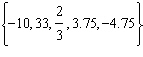a.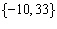b.c.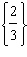d.e. none are rational

2.

Find a true inequality that involves the real number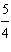.
 a.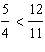b.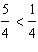c.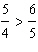d.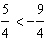e.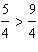3.

Evaluate: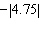a. –4.75 b. 4.75 c. 0.75 d. –0.75 e. 0

4.

Which of the following is an example of a rational number that is not a natural number?
 a. –9 b.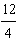c.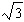d. 9 e.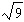5.

Find the sum.a. 0 b. 2 c. –2 d. –1 e. 1

6.

Find the difference.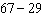a. 38 b. 28 c. 48 d. 37 e. 33

7.

Find the difference.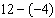a. 8 b. 9 c. –16 d. –8 e. 16

8.

Find the difference.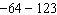a. –197 b. 59 c. 187 d. –187 e. –59

9.

A man awoke to a temperature of 40°F. By the time he arrived at work, the temperature had increased by 11°F. At lunch he noted the temperature had decreased from the temperature when he arrived at work by 12°F. Upon arriving home after work, the temperature had decreased another 13°F. What was the temperature when the man arrived home?
 a. 76°F b. 26°F c. 52°F d. 15°F e. 4°F

10.

Find the product.a. 5 b. 144 c. –1152 d. 1152 e. –144

11.

Use the vertical multiplication algorithm to find the product.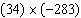a. 9,722 b. –249 c. –9,722 d. 9,622 e. –9,622

12.

Use the long division algorithm to find the quotient.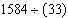a. 48 b. 33 c. 43 d. 53 e. 38

13.

Multiply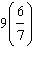and write the result in simplest form.
 a.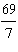b.c.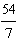d.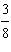e.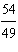14.

Multiply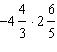and write the result in simplest form.
 a.b.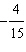c.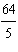d.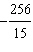e.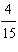15.

Divide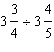and write the result in simplest form.
 a.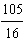b.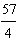c.d.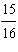e.16.

Write the fractionin decimal form.
 a. 0.6 b. 0.2 c. 9.9 d. 9.4 e. 0.1

17.

Evaluate the expression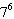.
 a.b.c.d.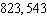e.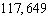18.

Evaluate the expression.
 a.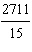b.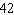c.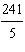d.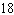e.19.

Identify the property of real numbers illustrated by the statement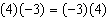.
 a. Commutative Property of Multiplication b. Commutative Property of Addition c. Distributive Property d. Associative Property of Addition e. Associative Property of Multiplication

20.

Rewrite the expression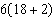by using the Distributive Property of real numbers.
 a.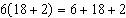b.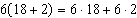c.d.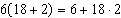e.